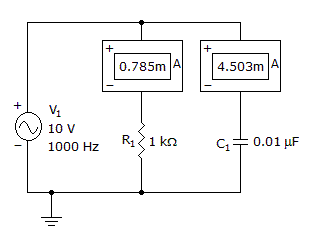# Electronics - Capacitors

### Exercise :: Capacitors - General Questions

11.

A capacitor's rate of charge is considered:

 A. magnetic B. a current block C. linear D. exponential

Explanation:

No answer description available for this question. Let us discuss.

12.

A resistor and an output capacitor form a series circuit with a square wave applied. The circuit is:

 A. an integrator B. a differentiator C. a multiplier D. a divider

Explanation:

No answer description available for this question. Let us discuss.

13.

If an open capacitor is checked with an ohmmeter, the needle will:

 A. stay on zero B. stay on infinity C. move from zero to infinity D. move from infinity to zero

Explanation:

No answer description available for this question. Let us discuss.

14.

What is the circuit's total current?A. 4.57 mA B. 9.327 mA C. 11.570 mA D. 13.830 mA

Explanation:

No answer description available for this question. Let us discuss.

15.

As a capacitor is being charged, current flowing into the capacitor will:

 A. increase B. decrease C. remain the same D. cannot tell